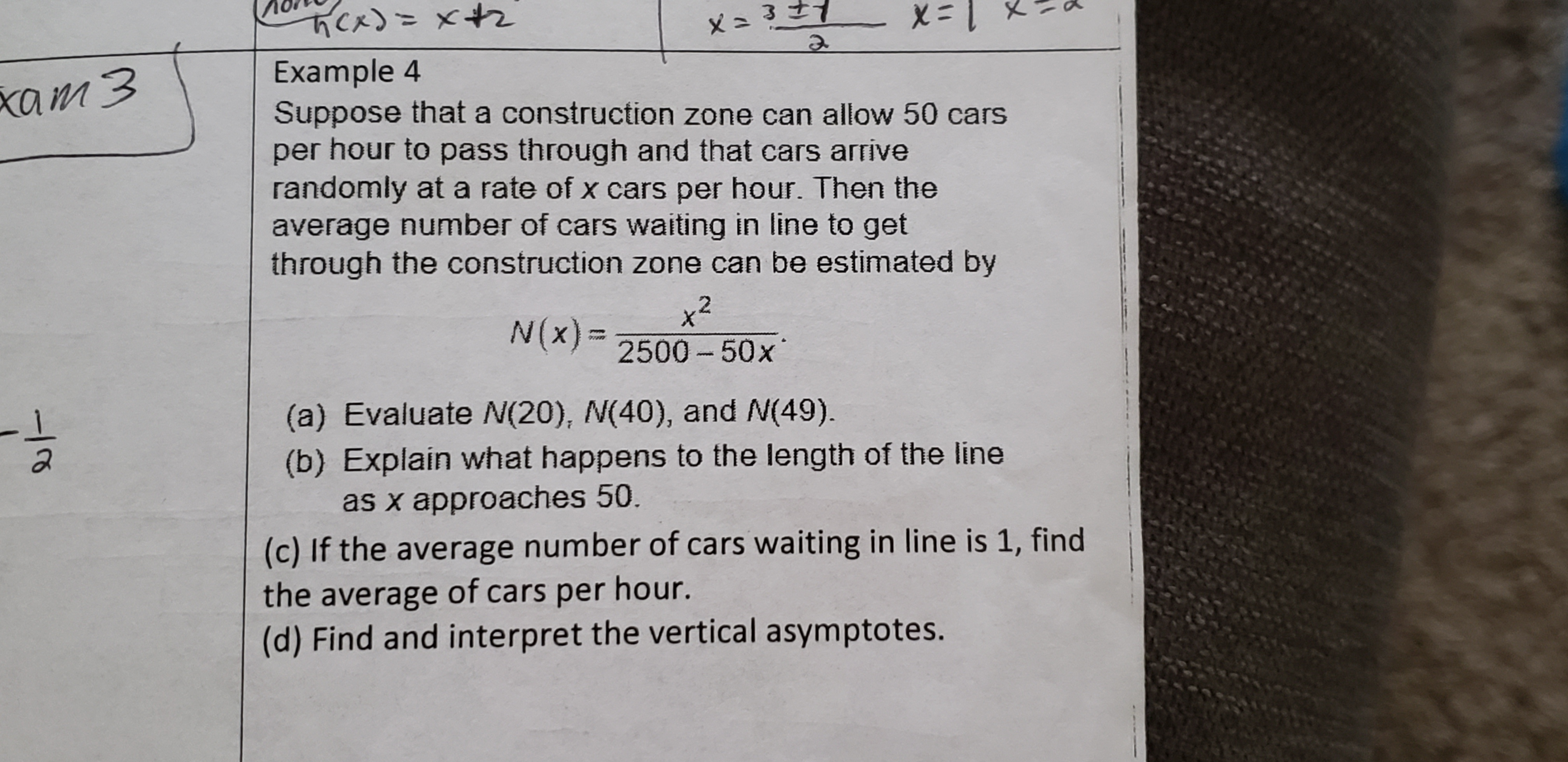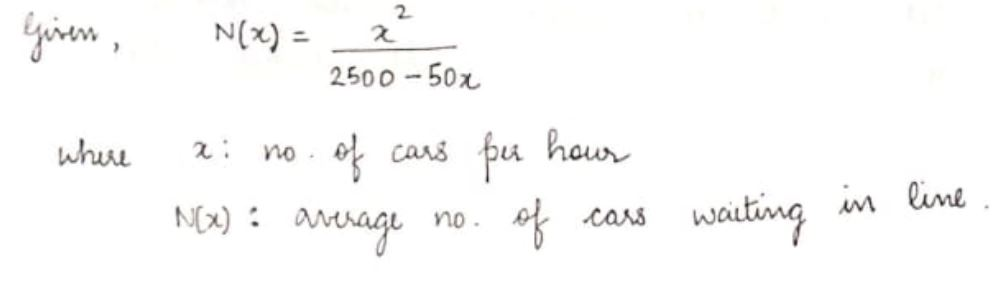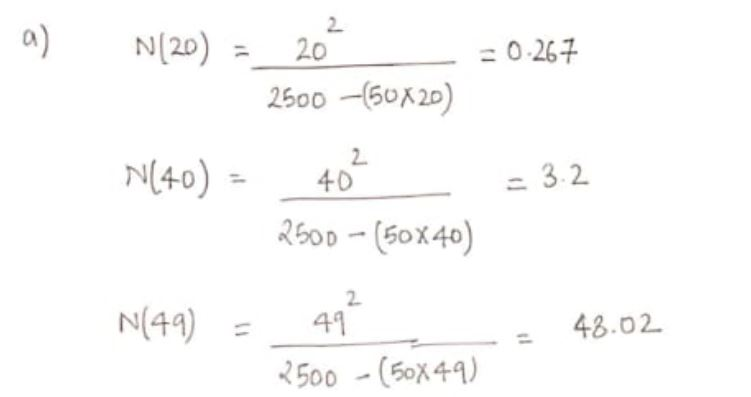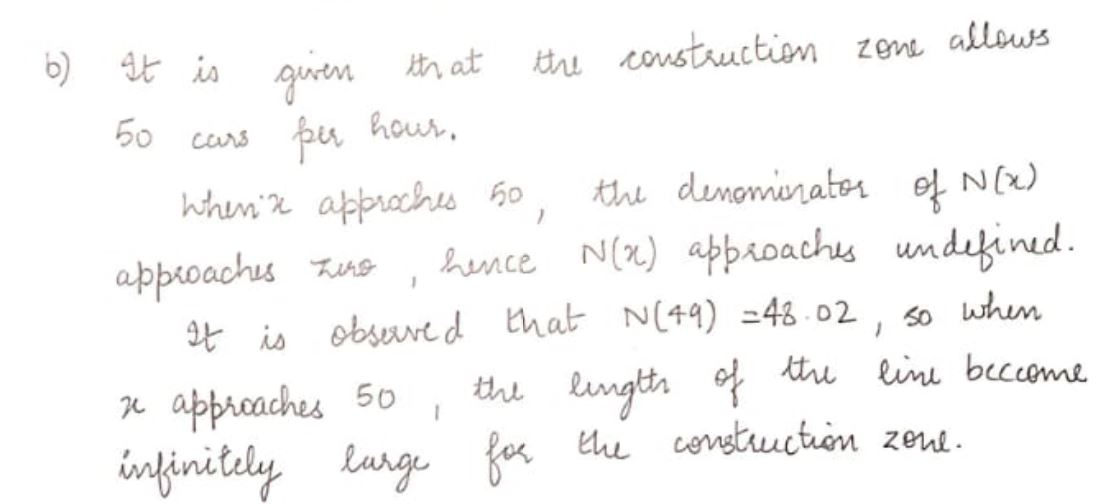# Cx)=x+2こメ 1=xExample 4Suppose that a construction zone can allow 50 carsper hour to pass through and that cars arriverandomly at a rate of x cars per hour. Then theaverage number of cars waiting in line to getthrough the construction zone can be estimated byxam3x²2500 - 50xN(x) =(a) Evaluate N(20), N(40), and N(49).(b) Explain what happens to the length of the lineas x approaches 50.(c) If the average number of cars waiting in line is 1, findthe average of cars per hour.(d) Find and interpret the vertical asymptotes.

Question
3 views

Liok at the attached photo pleasehelp_outlineImage TranscriptioncloseCx)=x+2 こメ 1=x Example 4 Suppose that a construction zone can allow 50 cars per hour to pass through and that cars arrive randomly at a rate of x cars per hour. Then the average number of cars waiting in line to get through the construction zone can be estimated by xam3 x² 2500 - 50x N(x) = (a) Evaluate N(20), N(40), and N(49). (b) Explain what happens to the length of the line as x approaches 50. (c) If the average number of cars waiting in line is 1, find the average of cars per hour. (d) Find and interpret the vertical asymptotes. fullscreen
check_circle

Step 1Step 2Step 3...

### Want to see the full answer?

See Solution

#### Want to see this answer and more?

Solutions are written by subject experts who are available 24/7. Questions are typically answered within 1 hour.*

See Solution
*Response times may vary by subject and question.
Tagged in